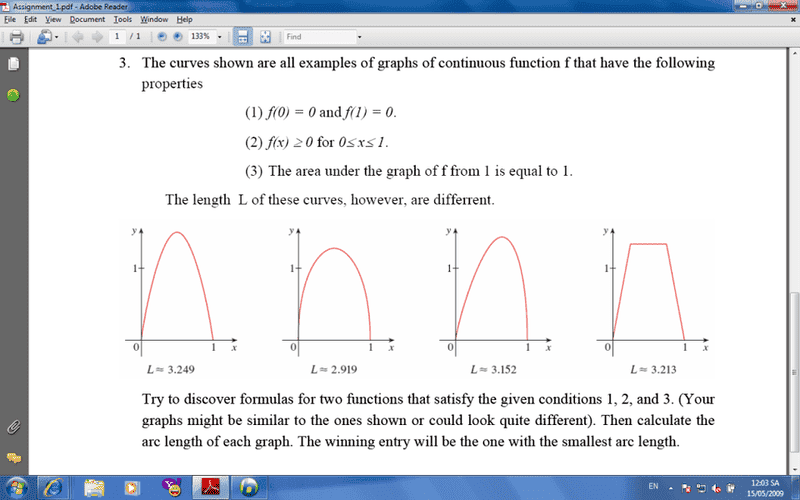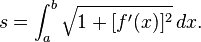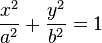• indifferent_2

#### indifferent_2

I have a quiz and I don't know how to solve this question ? Help meThis is my first post . I don't know where to post this topic . Can you move this topic into right place , Mod ?

For my money, the best you can do is an ellipse. You're going to have to fit it to make it work, though. I tried it, and my answer was better than any of the ones up there.

I think this should probably go in the HW section.

I've moved this to the PreCalculus Homework section because, although it mentions "area under the curve", calculus is really necessary.

The simplest graphs, I think, would be would be triangles. The area of a triangle with base b and height h is (1/2)bh. You want that to be equal to 1 and the base is 1 so the height h is easy to find, right? Just draw a straight line from (0,0) to that height for some x between 0 and 1, then a line from that point down to (1,0). The formula for that will be "piece-wise linear".

I have a quiz and I don't know how to solve this question ? Help meThis is my first post . I don't know where to post this topic . Can you move this topic into right place , Mod ?

Triangle or trapezoid is simplest. I'm not sure if a rectangle would satisfy the conditions.

I believe that, technically speaking, a rectangle would not constitute a *function*.

But a trapezoid, with inward leaning sides, would.

I believe that, technically speaking, a rectangle would not constitute a *function*.
No. Perhaps not, but what about the function f(x) = 8 ?
Is that not a rectangle function on at clearly defined area, like this?

So a rectangle, in this respect ,might be considered a function,
howewer for this exercise, not the correct one, as f(x)
needs to be 0 both at x=0 and x=1.

Anyway, you could use absolute value functions to take care of this problem.
I'd go with that.

Maybe integration can give other possible solutions?

Last edited:
No. Perhaps not, but what about the function f(x) = 8 ?
Is that not a rectangle function on at clearly defined area, like this?

So a rectangle, in this respect ,might be considered a function,
howewer for this exercise, not the correct one, as f(x)
needs to be 0 both at x=0 and x=1.

Anyway, you could use absolute value functions to take care of this problem.
I'd go with that.

Maybe integration can give other possible solutions?
No, it is not. f(x)= 8 is a constant function, its graph is a straight line, even on [0,1]. A "rectangle" would have, as part of its graph, vertical sides and would not be the graph of a function.

No, it is not. f(x)= 8 is a constant function, its graph is a straight line, even on [0,1]. A "rectangle" would have, as part of its graph, vertical sides and would not be the graph of a function.

Remember that Df for f(x) is [0,1]. So the sides are there, as given by condition 2), the latter part...But don't let's argue. I will give you right in the part of sides on a rectangle. Usually there are 4 of them..

Last edited:
larstuff:

A rectangle has four sides. The function f(x)=8 does not constitute a rectangle on any domain. What you're talking about is a constant function. The area of integration will certainly be a rectangle, but that's a whole other story.

Like I said, I bet an ellipse will win you the points.

hix . many idea ! Can u give me a complete solution ? I have 28 hours to do this quiz .:((
Thanks 4 many helps .

Last edited:
larstuff:

A rectangle has four sides. The function f(x)=8 does not constitute a rectangle on any domain. What you're talking about is a constant function. The area of integration will certainly be a rectangle, but that's a whole other story.

Like I said, I bet an ellipse will win you the points.

There seems to be a problem with the cut ellipsis too, I'm afraid. The equation for calculating arc length is given by the integral formula:So when trying to calculate this integral, we get similar problems to those of the rectangle.

All ellipsis functions derived from simple manipulation of the ellipsis formula:that are divided symmetrically by the x-axis and are defined in such a way that they start off in the point [0,0] and end in [0,1] have no distinct derivative values at their endpoints, simply because the curve's slope approches (+/-)infinity exactly there. Thus the arc length cannot be computed using the formula above.

I fully agree that the ellipsis is the best bet for a function with the shortest arc length, it is a squeezed circle and therefore should have the shortest possible arc length. But as the derivatives are not defined at the endpoints and the enpoints are included in the Df of the function, such ellipses as described above are not functions on this domain.

Of cource, we could sink the ellipses with an infinitesimal parallell to the y-axis. But an infinitesimal is very diffucult to compute numerically. So, in my book the problem, as presented here, is a paradox.

Of cource I could be wrong in this. There are ways of finding the circumference of an ellipse. But if You look this up, interestingly, You find that this circumference is described as an infinite series. True, you can make almost perfect approximations of the circumference, but still, they will not give you an exact number, as the number is irrational.

Have a look at the wikipedia article on this, if You like:

http://en.wikipedia.org/wiki/Ellipse

And indifferent 2, I could give You the function of the ellipsis that has exactly 1 as the area under the graph, with illustrations and explanations, but, as I have argued above, the ellipsis will not satisfy all the conditions in the exercise, as no exact arc length can be computed, alass.

Last edited:
can u give me a complete answer for this quiz, larstuff ? I'm mad of it . Thanks 4 help :D

can u give me a complete answer for this quiz, larstuff ? I'm mad of it . Thanks 4 help :D

The function for the ellipse is:

f(x)= 4/pi * sqrt ( 1 + ((x - 0,5)/0,5)^2 )

The TEX on these pages is difficult to get ...

But again, though this is the correct function for the semi-ellipse between x=0 and x=1,
with area exactly 1, you will get a hard time computing the arc length, by the formula above.

One could argue that the arc length is half of the perimeter of the ellipse that this function is built on.
The numerical value of this length is about 2,9194626481. I think this is indicated by fig.2 above, although
the rounding below the graph must be wrong, as an arc length of 2.91 never can hold an area of 1 between 0 and 1, if
AUMathTutor's advice holds true, and I think it does.

This ellipse spans from x=0 to x=1 and from y= -4/pi to y= 4/pi , and therefore
it must have an area of exactly 1, as this area always is pi x a x b, where a=0,5 and b=4/pi .
This gives the whole ellipse an area of 2, and half an ellipse the area of 1.

The formula for the ellipse is:

((x-0,5)/0,5)^2 + (y/(4/pi))^2 = 1 | Tex problems, sorry again

Solving this for y, you will find the above function.

Hope this helps. I will have to get some sleep now.

Last edited:
Can u explaint more carefully , how to find this func ? thanks u

Oh . Thanks u a lot .

larstuff:

The semi-ellipse is a function on [0,1] constitutes a function since for any x in [0,1] there corresponds exactly one real number related to x. It passes the vertical line test.

The arc length will have to be approximate. I think you'll find the ellipse is the second graph in the figure.

Other ideas? Any function of the form

x^a - x^b, with a and b greater than zero

Will satisfy the condition that f(0) = f(1) = 0.